Include Top

# Attribute P ARL

1. Click SigmaXL > Templates & Calculators > Control Chart Templates > Average Run Length (ARL) Calculators >Attribute P ARL. This template is also located at SigmaXL > Control Charts > Control Chart Templates> Average Run Length (ARL) Calculators >Attribute P ARL.

2. The default template settings are Specify = Exact (Test 1 Only), Subgroup Size = 50, In-Control Historical Proportion (CL) = 0.5.Notes: Specify Exact (Test 1 Only) or Monte Carlo using the drop-down list. Parameters to be specified will be shown in yellow highlight, otherwise they are hidden. Exact uses the binomial cumulative distribution function. Monte Carlo simulation uses binomial random data with specified proportion and allows you to assess the ARL performance of all 4 Tests for Special Causes. Test 1 - 1 point more than 3 standard deviations from the center line (CL) is always applied. Monte Carlo simulation also produces the table of Run Length Standard Deviation and Percentiles (scroll right to view).

3. Click the Calculate Attribute P ARL button to reproduce the ARL table and chart.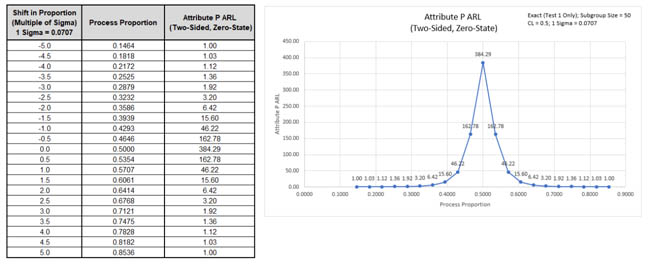The ARL0 (in-control ARL with 0 sigma shift in proportion) for the Attribute P chart is 384.29. The ARL1 for a small 1 sigma shift is 46.22, so is slow to detect. On the other hand, a large 3 sigma shift in proportion has an ARL = 1.92, so is detected rapidly.

Note: The Process Proportion values are displayed on the ARL chart, but the shift in proportion as a multiple of sigma is also included in the table.

4. Now we will use Monte Carlo simulation to obtain approximate Run Length standard deviation and percentiles for the Attribute P chart. Select Specify = Monte Carlo. Enter Subgroup Size = 50, In-Control Historical Proportion (CL) = 0.5, Number of Replications = 1e4, Test 2 = N/A, Test 3 = N/A, Test 4 = N/A.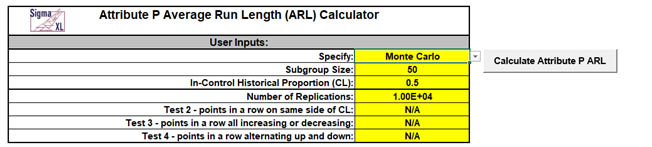5. Click the Calculate Attribute P ARL button to produce the Monte Carlo approximate ARL table, ARL chart and Run Length Standard Deviation and Percentiles table (scroll right to view). Monte Carlo simulation with 10,000 (1e4) replications will take about a minute to run.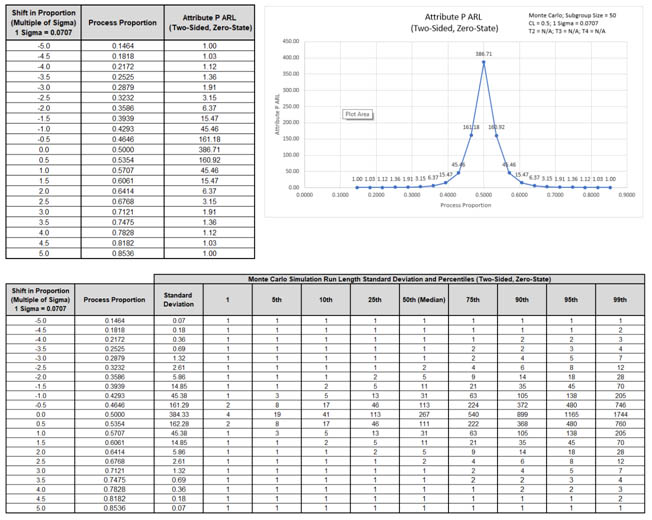The additional run length statistics show the large variation of run length values. The MRL0 = 267 (in-control median run length with 0 sigma shift in process proportion).

Note: The results will vary slightly since this is Monte Carlo simulation.

6. Now we will use Monte Carlo simulation to assess the Attribute P chart with all 4 Tests for Special Causes applied. Enter Specify = Monte Carlo, Subgroup Size = 50, In-Control Historical Proportion (CL) = 0.5, Number of Replications = 1e4, Test 2 = 9, Test 3 = 6, and Test 4 = 14.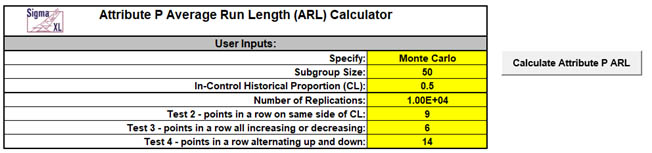Note: These are the test settings used as defaults in SigmaXL > Control Charts > ‘Tests for Special Causes’ Defaults. Test 1 is always applied.

7. Click the Calculate Attribute P ARL button to produce the Monte Carlo approximate ARL table, ARL chart and Run Length Standard Deviation and Percentiles table: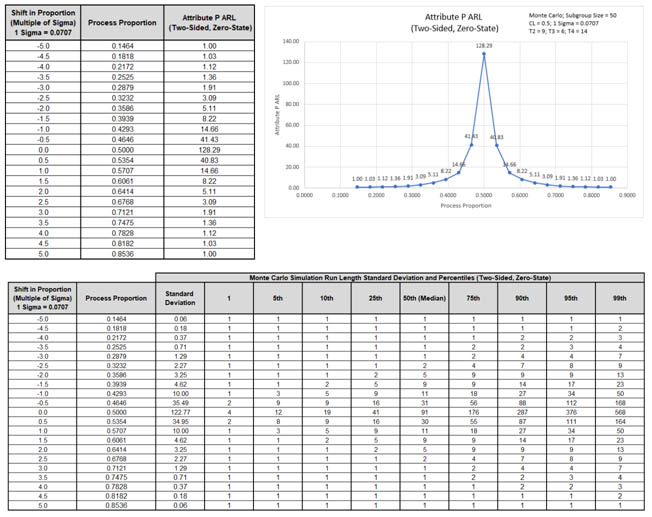ARL0 with all 4 tests for special causes is approx. 128.3. This is a poor performance with a 3 x increase (384.3/128.3) in false alarms compared to Exact Test 1 only. MRL0 is approx. 91. On the other hand, ARL1 for a small 1 sigma shift in proportion is approx. 14.7, so is much faster to detect than the Exact Test 1 only ARL1 of 46.22.

8. We will now assess ARL for a low in-control proportion value. Enter Specify = Exact (Test 1 Only), Subgroup Size = 50, In-Control Historical Proportion (CL) = 0.1.9. Click the Calculate Attribute P ARL button to produce the ARL table and chart for these settings: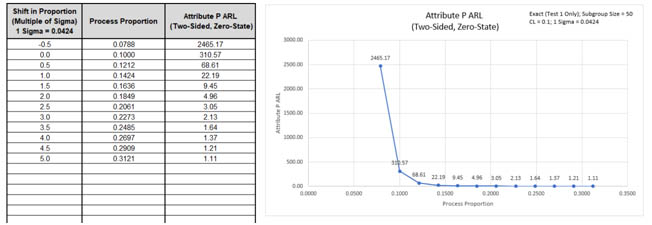The ARL0 for the Attribute P chart is 310.57. The ARL1 for a small positive 1 sigma shift in proportion is 22.19, so is slow to detect. On the other hand, a large positive 3 sigma shift in proportion has an ARL = 2.13, so is detected rapidly. The ARL for a small negative 0.5 sigma shift in proportion is 2465.7 so negative shifts cannot be detected with these settings.

Note: The P Chart LCL is 0 for this CL value.

10. Now we will use Monte Carlo simulation to assess ARL for a low in-control proportion value but with all 4 Tests for Special Causes applied. Enter Specify = Monte Carlo, Subgroup Size = 50, In-Control Historical Proportion (CL) = 0.1, Number of Replications = 1e4, Test 2 = 9, Test 3 = 6, and Test 4 = 14.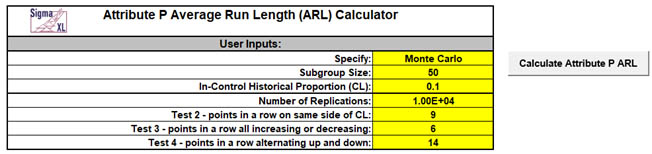11. Click the Calculate Attribute P ARL button to produce the ARL table and chart for these settings.ARL0 with all 4 tests for special causes is approx. 90.5. This is a poor performance with a 3.4 x increase (310.6/90.5) in false alarms compared to Exact Test 1 only. On the other hand, ARL1 for a small 1 sigma shift in proportion is approx. 12.6, so is much faster to detect than the Exact Test 1 only ARL1 of 22.19. Also, it is now possible to detect a negative one or two sigma shift in proportion.

12. We will now assess ARL for a low in-control proportion value but with larger subgroup size. Enter Specify = Exact (Test 1 Only), Subgroup Size = 100, In-Control Historical Proportion (CL) = 0.1.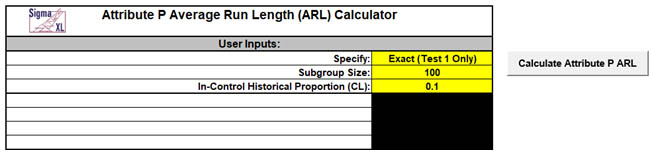13. Click the Calculate Attribute P ARL button to produce the ARL table and chart for these settings:mean is 31.31, so is slow to detect. On the other hand, a large positive 3 sigma shift in proportion has an ARL = 2.28, so is detected rapidly. Small negative shifts still cannot be detected, but a large negative 3 sigma shift in proportion can be detected with ARL = 2.73.

14. Now we will use Monte Carlo simulation to assess ARL for a low in-control proportion value with subgroup size = 100, but with all 4 Tests for Special Causes applied. Enter Specify = Monte Carlo, Subgroup Size = 100, In-Control Historical Proportion (CL) = 0.1. Number of Replications = 1e4, Test 2 = 9, Test 3 = 6, and Test 4 = 14.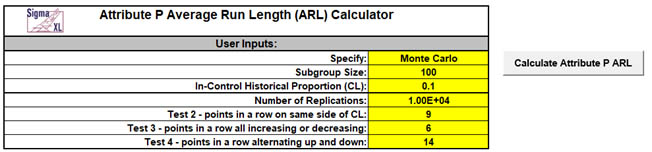15. Click the Calculate Attribute P ARL button to produce the ARL table and chart for these settings: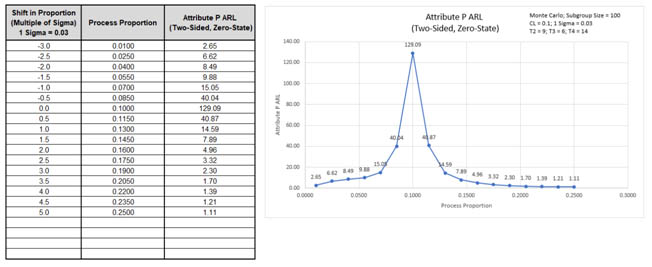ARL0 with all 4 tests for special causes is approx. 129.1. This is a poor performance with a 3.9 x increase (498.7/129.1) in false alarms compared to Exact Test 1 only. On the other hand, ARL1 for a small 1 sigma shift in proportion is approx. 14.6, so is much faster to detect than the Exact Test 1 only ARL1 of 31.31. Also, it is now possible to detect a negative one, two or three sigma shift in proportion.

# Template Notes:

1. Specify Exact (Test 1 Only) or Monte Carlo using the drop-down list. Parameters to be specified will be shown in yellow highlight, otherwise they are hidden.
2. Exact uses the binomial cumulative distribution function. Monte Carlo simulation uses binomial random data with specified proportion and allows you to assess the ARL performance of all 4 Tests for Special Causes. It also produces the table of Run Length Standard Deviation and Percentiles (scroll right to view).
3. Test 1 - 1 point more than 3 standard deviations from the center line (CL) is always applied.
4. Enter the Subgroup Size.
5. Enter the In-Control Historical Proportion (CL). This is a value between 0 and 1.
6. If applicable, enter Number of Replications. 1000 (1e3) replications will be fast, approx. 10 seconds, but will have an ARL0 error approx. = +/- 10%; 10,000 (1e4) replications will take about a minute, with an ARL0 error = +/- 3.2%; 100,000 (1e5) replications will take about ten minutes, with an ARL0 error = +/- 1%.
7. If applicable, select values for Tests 2 to 4 using the drop-down list. "N/A" indicates that the test is not applied. Tests 2 and 3 provide options that match those provided in SigmaXL's 'Tests for Special Causes' Defaults dialog.
8. Click the Calculate Attribute P ARL button to produce the ARL table and chart. If Monte Carlo was selected, the table of Run Length Standard Deviation and Percentiles will also be produced.
9. The Attribute P ARL is for a two-sided chart with zero-state, i.e., the shift is assumed to occur at the start. The proportion is also assumed to be known. This will not likely be the case in use, but is still useful for determining parameter settings and comparison of ARL across chart types.
10. The ARL Chart is similar to an Operating Characteristic (OC) Curve, except that the Y axis is ARL rather than Beta probability. Note that the P chart may be ARL biased, with maximum ARL occurring above or below the CL.
11. Due to the complexity of calculations, SigmaXL must be loaded and appear on the menu in order for this template to function. Do not add or delete rows or columns in this template.

# REFERENCES:

1. Montgomery, D.C. (2013), Introduction to Statistical Quality Control, Seventh Ed., Wiley.

# Web Demos

Our CTO and Co-Founder, John Noguera, regularly hosts free Web Demos featuring SigmaXL and DiscoverSim